Courses

# Test: CPU & I/O Scheduling- 4

## 20 Questions MCQ Test Operating System | Test: CPU & I/O Scheduling- 4

Description
This mock test of Test: CPU & I/O Scheduling- 4 for Computer Science Engineering (CSE) helps you for every Computer Science Engineering (CSE) entrance exam. This contains 20 Multiple Choice Questions for Computer Science Engineering (CSE) Test: CPU & I/O Scheduling- 4 (mcq) to study with solutions a complete question bank. The solved questions answers in this Test: CPU & I/O Scheduling- 4 quiz give you a good mix of easy questions and tough questions. Computer Science Engineering (CSE) students definitely take this Test: CPU & I/O Scheduling- 4 exercise for a better result in the exam. You can find other Test: CPU & I/O Scheduling- 4 extra questions, long questions & short questions for Computer Science Engineering (CSE) on EduRev as well by searching above.
QUESTION: 1

### A computer has six tape drives, with n processes competing for them. Each process may need two drives. What is the maximum value of n for the system to be deadlock free?

Solution:

Given tape drive = 6 and each process may need 2 drive. When we give 1 drive to 1 process then total process will be 6 but in this case there will definitely deadlock occur because every process contain 1 drive and waiting for another drive which is hold by other process therefore when we reduce 1 process then system to be deadlock free. Hence maximum value of n = 6 - 1 = 5. Option (B) is correct.

QUESTION: 2

### Each Process Pi, i= 1.......9 is coded as follows repeat  P(mutex) {Critical section} V(mutex) forever The code for P10 is identical except it uses V(mutex) in place of P(mutex). What is the largest number of processes that can be inside the critical section at any moment?

Solution:

Consider code for i= 1 to 9 Initially mutex value set to 1, so it can allow only 1 process at a time. Now p1 enter into critical section and by this time remaining all are in block state. (i.e blocked processes= 2,3,4,5,6,7,8,9). But consider code for 10th process, it tells that unblock any process because it contain operation. Because of this it can unblock the processes and send it to the critical section. By doing this all processes can enter into critical section. So, finally there are 10 processes at maximum can enter into critical section.

Alternative Way- There is loop which runs forever So P10 runs forever. And it has capability to put all other processes to Critical Section. Moreover they Asked Maximum Number of process that can be Present at critical Section So Answer is 10. Option (D) is correct.

QUESTION: 3

### A critical section is a program segment

Solution:

Critical section is the part of program where shared resources are accessed by process.It also contain shared variable or shared data and rest portion of the program is called non-critical section or remaining section.

Option (C) is correct.

QUESTION: 4

A system shares 9 tape drives. The current allocation and maximum requirement of tape drives for 4 processes are shown below: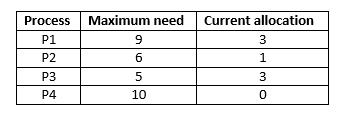Which of the following best describes the current state of the system?

Solution:

There are 9 tapes and allocated (3+1+3+0 =) 7, so remaining available tapes are 9 - 7 = 2.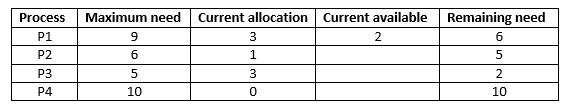Now we can satisfies only process P3 first which require only 2 tapes, then current available will be total 5. Similarly, we can satisfies only process P2 which require only 5 tapes, then current available will be total 6. Then, we can satisfies only process P1 which require only 6 tapes, then current available will be total 9. But we can not satisfied remaining need of process P4, because it requires 10 tapes and remaining need 9.

Option (C) is correct.

QUESTION: 5

A solution to the Dining Philosophers Problem which avoids deadlock is:

Solution:

In Dining Philosophers Problem it ensure that one particular philosopher picks up the left fork before the right fork, and that all other philosophers pick up the right fork before the left fork which avoids the deadlock condition. Option (C) is correct.

QUESTION: 6

Consider the following solution to the producer-consumer synchronization problem. The shared buffer size is N. Three semaphores empty, full and mutex are defined with respective initial values of 0, N and 1. Semaphore empty denotes the number of available slots in the buffer, for the consumer to read from. Semaphore full denotes the number of available slots in the buffer, for the producer to write to. The placeholder variables, denoted by P, Q, R and S, in the code below can be assigned either empty or full. The valid semaphore operations are: wait() and sigmal().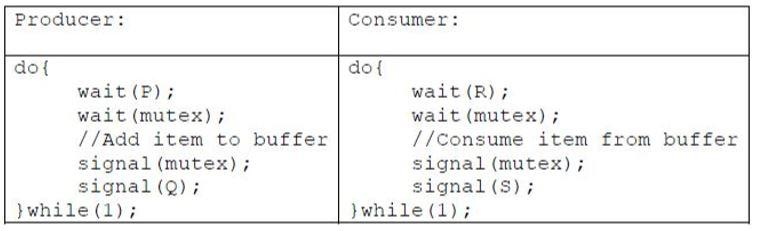Which one of the following assignments to P, Q, R and S will yield the correct solution?

Solution:

Given, Empty = 0 Full = N Mutex = 1 Since value of empty semaphore is 0, so you can not wait empty semaphore in first attempt. Note - empty semaphore denotes the number of filled slots, so producer process must deal with empty and mutex semaphores. full semaphore denotes the number of empty slots so consumer process must deal with full and mutex semaphores. Option (A) causes starvation. OPtion (B) causes starvation. Option (D) since number of filled slots are 0 initially denoted by empty semaphore, so consumer process can not consume. So, this implimentation is wrong. Only P: empty, Q: full, R: full, S: empty is correct order to ensure deadlock-free and starvation free implementation. Option (C) is correct.

QUESTION: 7

Consider a system with 3 processes that share 4 instances of the same resource type. Each process can request a maximum of K instances. Resource instances can be requested and released only one at a time. The largest value of K that will always avoid deadlock is _______ .

Note - This was Numerical Type question.

Solution:

Given, Number of processes (P) = 3 Number of resources (R) = 4 Since deadlock-free condition is:
R ≥ P(N − 1) + 1
Where R is total number of resources, P is the number of processes, and N is the max need for each resource.
4 ≥ 3(N − 1) + 1
3 ≥ 3(N − 1)
1 ≥ (N − 1)
N ≤ 2
Therefore, the largest value of K that will always avoid deadlock is 2. Option (B) is correct.

QUESTION: 8

Which of the following is not true with respect to deadlock prevention and deadlock avoidance schemes ?

Solution:

In Deadlock Prevention mechanism a set of methods are devised to ensure at least one of the necessary conditions "Mutual Exclusion", "Hold and Wait", "Circular Wait", "Non-preemption" can not hold. Option (A) is correct.

QUESTION: 9

In a system, there are three types of resources: E, F and G. Four processes P0, P1, P2 and P3 execute concurrently. At the outset, the processes have declared their maximum resource requirements using a matrix named Max as given below. For example, Max[P2, F] is the maximum number of instances of F that P2 would require. The number of instances of the resources allocated to the various processes at any given state is given by a matrix named Allocation. Consider a state of the system with the Allocation matrix as shown below, and in which 3 instances of E and 3 instances of F are the only resources available.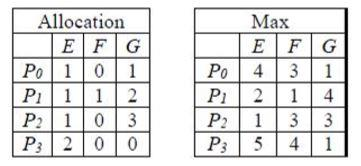From the perspective of deadlock avoidance, which one of the following is true?

Solution: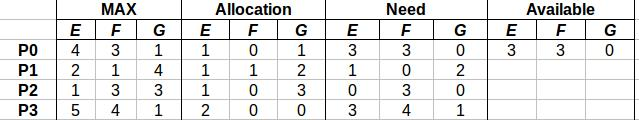Available (3, 3, 0), which can satisfy either P0 or P2. Take P0 <3, 3, 0>.

After completion we have (3, 3, 0) + (1, 0, 1) = (4, 3, 1) Take P2 <0, 3, 0>.

After completion we have (4, 3, 1) + (1, 0, 3) = (5, 3, 4) Take P1 <1, 0, 2>.

After completion we have (5, 3, 4) + (1, 1, 2) = (6, 4, 6) Take P3 <3, 4, 1>.

After completion we have (6, 4, 6) + (2, 0, 0) = (8, 4, 6)

Safe sequence : P0→P2→P1→P3 Therefore, option (A) is Correct.

QUESTION: 10

Which of the following is not true with respect to deadlock prevention and deadlock avoidance schemes?

Solution:

Deadlock Prevention: Deadlocks can be prevented by preventing at least one of the four required

conditions:

1. Mutual Exclusion – not required for sharable resources; must hold for non-sharable resources.

2. Hold and Wait – must guarantee that whenever a process requests a resource, it does not hold any other resources. Require process to request and be allocated all its sources before it begins execution, or allow process to request resources only when the process has none. Low resource utilization; starvation possible. Restrain the ways request can be made.

3. No Pre-emption – If a process that is holding some resources requests another resource that cannot be immediately allocated to it, and then all resources currently being held are released. Pre-empted resources are added to the list of resources for which the process is waiting. Process will be restarted only when it can regain its old resources, as well as the new ones that it is requesting.

4. Circular Wait – impose a total ordering of all resource types, and require that each process requests resources in an increasing order of enumeration.

QUESTION: 11

Solution:

There are 4 conditions required for a deadlock to occur: 1) Mutual Exclusion 2) No pre-emption 3) Hold and wait 4) Circular wait When there is only one resource, the conditions of hold and wait and circular wait get eliminated. With the assumption that no process can use a resource for infinite amount of time, whenever a process will finish its execution , another process can get the resource. So a deadlock can't occur. So, option (D) is correct.

QUESTION: 12

Which of the following is not a necessary condition for deadlock?

Solution:

There are 4 necessary conditions for Deadlock to occur:

1) Mutual Exclusion

2) No pre-emption

3) Hold and Wait

4) Circular wait

Option (B) is correct.

QUESTION: 13

What is the minimum number of resources required to ensure that deadlock will never occur, if there are currently three processes P1, P2 and P3 running in a system whose maximum demand for the resources of same type are 3, 4, and 5 respectively.

Solution:

let the resources needed by P1 = R1 =3, the number of resources needed by P2 = R2 = 4 and so on.. Minimum resources required to ensure that deadlock will never occur = (R1-1) + (R2 1) + (R3-1) + 1 = (3-1) + (4-1)+ (5-1) + 1 = 10. Option (D) is correct.

QUESTION: 14

A total of 9 units of a resource type available, and given the safe state shown below, which of the following sequence will be a safe state?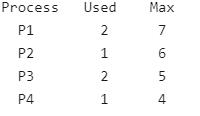Solution:

Applying Banker’s Algorithm, Need matrix of the processes are:
Process   Used    Max    Need
P1              2         7        5
P2              1         6        5
P3              2         5        3
P4              1         4        3
Currently Available resources = Available - Allocated resources = 9 - 6 = 3 If the request of P4 is granted first then it would release a maximum of 4 resources after its execution, and if P1 or P2 are allocated next then their requests can not be fulfilled as both of them require 5 resources each. So, that eliminates Options (A), (B) and (C). Option (D) is correct.

QUESTION: 15

When a process is rolled back as a result of deadlock the difficulty which arises is

Solution:

When a process is rolled back as a result of deadlock, the difficulty which arises is starvation because when we rollback the process then there may be possibility that again deadlock occur after that again process will wait for indefinite amount of time that’s why it goes to starvation. On the other hand Low device utilization and cycle stealing is not a related concept to the rollback of process as a result of deadlock. Option (A) is correct.

QUESTION: 16

Consider a system having "n" resources of same type. These resources are shared by 3 processes, A, B, C. These have peak demands of 3, 4, and 6 respectively. For what value of "n" deadlock won't occur

Solution:

The value of n for which the deadlock can't occur = n(k-1) + 1 Number of min resources required = (3-1) + (4-1) + (6-1) + 1 = 11 So, option (D) is correct.

QUESTION: 17

In which of the following four necessary conditions for deadlock processes claim exclusive control of the resources they require?

Solution:

Mutual Exclusion is a condition when one or more than one resource are non-sharable (Only one process can use at a time) i.e. processes claim exclusive control of the resources they require. So, option (B) is correct.

QUESTION: 18

Consider a system having m resources of the same type. These resources are shared by 3 processes A, B, C which have peak time demands of 3, 4, 6 respectively. The minimum value of m that ensures deadlock will never occur is

Solution:

Let the resources needed by P1 = R1 = 3, the number of resources needed by P2 = R2 = 4 and so on. Minimum resources required to ensure that deadlock will never occur = (R1-1) + (R2-1) + (R3-1) + 1 = (3-1) + (4-1) + (6-1) + 1 = 11 Option (A) is correct.

QUESTION: 19

A counting semaphore was initialized to 10. Then 6 P (wait) operations and 4 V (signal) operations were completed on this semaphore. The resulting value of the semaphore is

Solution:

Initially we have semaphore value = 10 Now we have to perform 6 p operation means when we perform one p operation it decreases the semaphore values to one. So after performing 6 p operation we get, semaphore values = 10 - 6 = 4 and now we have to perform 4 v operation means when we perform one V operation it increases the semaphore values to one. So after performing 4 v operation we get, semaphore values = 4 + 4 = 8. Option (B) is correct.

QUESTION: 20

A system has n resources R0,...,Rn-1,and k processes P0,....Pk-1.The implementation of the resource request logic of each process Pi is as follows:
if (i % 2 == 0) {
if (i < n) request Ri
if (i+2 < n) request Ri+2
}
else {
if (i < n) request Rn-i
if (i+2 < n) request Rn-i-2
}
In which one of the following situations is a deadlock possible?

Solution:

No. of resources, n = 21
No. of processes, k = 12

Processes {P0, P1....P11}  make the following Resource requests:
{R0, R20, R2, R18, R4, R16, R6, R14, R8, R12, R10, R10}

For example P0 will request R0 (0%2 is = 0 and 0< n=21).

Similarly, P10 will request R10.

P11 will request R10 as n - i = 21 - 11 = 10.

As different processes are requesting the same resource, deadlock
may occur.